# Abstract

In this paper a study is performed on application of two recovery methods, i.e. Superconvergent Patch Recovery (SPR) and the Recovery by Equilibrium of Patches (REP), to plate problems. The two recovery methods have been recognized to give similar results in adaptive solutions of two dimensional stress problems. While the former applies a least square fit over a set of values at the so called superconvergent points, the latter does not need any knowledge of such points and thus has a wider application especially in non-linear problems. The formulation of REP is extended to Reissner-Mindlin plate problems. The convergence rates of the recovered fields of the gradients obtained from application of the two methods are compared using series of regular triangular and rectangular meshes for thick and thin plate solution cases. Assumed strain formulation based elements, i.e. the elements formulated by Mixed Interpolation of Tensorial Components, as well as conventional from of elements based on selective integration schemes are employed for the study.

In order to investigate the possibility of any improvement in the results by adding equilibrium constraints to SPR, as some authors suggest for simple two-dimensional problems, some weighted forms of such conditions are designed and added to the formulation. Comprehensive study has been given first by varying the weight terms to obtain the best enhanced results and then using the optimal values to investigate the effects of the constraints on the rate of convergence. It is observed that despite of the cost of this approach, due to the coupling of the gradient terms, no significant improvement is achieved.

Keywords: Recovery, Plate, Error Estimate, Superconvergent

# 1. INTRODUCTION

One important category of problems in engineering is of plates under lateral loads. Numerical solution of thin/thick plates has so far received a considerable attention by many researchers. Finite element method has been recognized as the most powerful numerical method for solution of this class of problems. To achieve a desired level of accuracy, adaptive procedures are usually employed. From two major parts of an adaptive finite element procedure, i.e. error estimation and mesh generation, here we focus on the first part.

For two dimensional problems, error estimation has now reached to a rather mature level and a rich literature is available for the subject. Two forms of approaches are sought by engineers. The first approach initiated by pioneering work due to Babuška and Rheinboldt is categorized as residual based error estimates . The second approach introduced by Zienkiewicz and Zhu, known as ZZ estimate, falls in the category of recovery based error estimates . For practical engineering problems, however, Babuška and co-workers, in a series of papers [3-5], showed that the most effective error estimates are those based on recovery methods, especially ZZ estimate utilizing Superconvergent Patch Recovery method (SPR) proposed in . Success of a recovery based estimate, as proven in , is in debt to superconvergent property of the recovery method. To construct a recovered gradient field exhibiting superconvergent property in SPR a polynomial is fitted over a set of sampling points known to be best in a patch of elements surrounding a node. For the choice of patches, around singularities and other special cases, the reader can consult to reference 8 for further details. Another superconvergent method, which was later introduced in  and called Recovery by Equilibrium in Patches (REP), basically utilizes patches of elements as well. The method uses a weighted form of equilibrium equations to produce the recovered fields of the gradients. The method was later improved, in , and proven to pass the robustness test introduced by Babuška and co-workers in [3-5]. Unlike the SPR method which needs some information for superconvergent points over the elements, REP does not require any knowledge of such points. A combined formulation was later given in  in which the functionals used in SPR and REP were added together in order to make use of advantages of both methods. Such combined method, however, still needs some information at sampling points.

Unlike simple two dimensional problems, few studies can be found for errors in finite element solutions using mixed formulations such as the one is used in Reissner-Mindlin theory. In the category of residual based error estimates, recent researches by Weinberg and Carstensen can be found in the literature [12,13]. In the category of recovery based error estimates, however, some more studies can be traced. The pioneering work is due to Zienkiewicz and Zhu  who used an early version of the estimator. Later in a study by Lee et al  the recovered fields of stresses were obtained by application of SPR procedure augmented with some equilibrium constraints. The methodology is similar to the ones employed by Wiberg and Abdulwahab [16,17], and Blacker and Belytschko  for two dimensional stress analyses.

In order to pave the way for application of recovery based error estimates to plate problems, some investigations were performed by Zhang [19,20] aiming at giving a mathematical proof for existence of superconvergent points in some linear rectangular plate elements. The study was performed on a type of four node elements introduced by Bathe and co-workers [21-23] using an approach somewhat similar to reference 5. An overall conclusion of the study is that superconvergent property exists, at least, for interior elements and thus application of SPR can be promising.

In a research by Lee and Hobbs, application of ZZ estimate using SPR was studied through an adaptive procedure . The elements employed in the study were those introduced by Huang and Hinton [25,26] and the recovery used was the augmented form of SPR suggested in . Similar study can be seen in  for shell problems using elements introduced by Sze .

In another study on plate problems by Okstad et al in , statically admissible fields of smoothed stresses were used in SPR procedure to recover the stresses. Using such statically admissible fields is, in fact, equivalent to strictly satisfy the equilibrium constraints which are usually added to the functional and thus the study can be considered as a special case for similar studies [15, 24, 27]. Another kind of recovery method suitable for application of ZZ estimate can be found in literature for shell problems .

In this paper two forms of studies are given to demonstrate the applicability of SPR and REP (original/improved) to plate problems. In one form of study we shall compare the results obtained from application of the methods to series of regular meshes of elements. This, of course, requires extension of REP formulation to plate problems. For the finite element solutions a set of assumed strain elements introduced in [21-23] are employed. Both thick and thin plate problems will be examined here.

In another form of study we shall investigate the possibility of any improvement of the results by adding some weighted equilibrium constraints to the original form of SPR. Several types of constraints, including those suggested in [15-18] , are tested. During our studies we shall also give an answer to the common question regarding the role of the weights of the constraints on the accuracy and the convergence rate of the solutions.

The layout of the paper is as follows. In the second section of the paper, the mathematical model of Reissner-Mindin plate theory is given. The finite element formulation is then described followed by the assumption made for the elements used. The recovery procedures are formulated in the third section during which we shall extend the formulation of REP to plate problems. In order to study the effects of adding constraints on the recoveries, the definitions are given in section four. In section five we shall present the numerical results for each part of the study using some benchmark problems. The conclusions are given as the last section of this paper.

# 2. THE MODEL PROBLEM

Plates are usually formulated as special cases of three-dimensional problems assuming specific variation for in-plane displacement components i.e.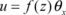, $v=f(z){\mbox{ }}{\theta }_{y}$
(1)

and a general form for the third one as

Where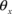and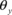are the components of the rotation vector of the mid-plane normal,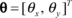, and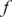is a suitable function indirection (see [31,32] for more recent formulations). In a Reissner-Mindlin type of formulation the variation of in-plane displacement is assumed to be linear in the transverse direction, i.e.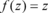, and the differences between the rotations of the mid-plane and those of its normal constitute the shear deformations. Thus

or in a more compact form;

The bending strains are evaluated from the rotation of the mid-plane i.e.

or in an operational form

 ${\epsilon }_{b}=z{\mbox{ }}{\boldsymbol {L}}{\mbox{ }}\theta \,=$$z{\mbox{ }}\kappa$ ,(6)

Stress-strain relations in a plate formulation are usually defined in a resultant from as

 ${\boldsymbol {M}}={\boldsymbol {D}}_{b}{\mbox{ }}\kappa$ , ${\boldsymbol {S}}={\boldsymbol {D}}_{s}{\mbox{ }}{\epsilon }_{s}$
(7)
 ${\boldsymbol {D}}_{b}={\frac {E{\mbox{ }}t^{3}}{12{\mbox{ }}{\mbox{ }}(1-{\nu }^{2})}}\left[{\begin{array}{ccc}1&\nu &0\\\nu &1&0\\0&0&\left(1-\nu \right)/2\end{array}}\right]$ , ${\boldsymbol {D}}_{s}={\frac {\alpha {\mbox{ }}E{\mbox{ }}t}{2{\mbox{ }}{\mbox{ }}(1+\nu )}}\left[{\begin{array}{cc}1&0\\0&1\end{array}}\right]$
(8)

Where E,,andare Young’s modulus, Poisson’s ratio, plate thickness and shear correction factor, respectively ( ${\textstyle \alpha ={\frac {5}{6}}}$ in this study). It should be noted that expressions (8) are obtained from a plane stress assumption made in Reissner-Mindlin formulation. More specifically, if one starts from (1) and (2) and uses a general three dimensional elasticity modulus, then the bending elasticity modulus will be different. In such a formulation a hierarchic model should be used instead of Reissner-Mindlin model. The reader may consult to reference 33 for more details.

The differential equilibrium equations may be written as:

Now substituting relations (4) and (7) into (9), the equilibrium equations may be written in terms of displacement components as

 ${\boldsymbol {L}}^{T}{\boldsymbol {D}}_{b}{\mbox{ }}{\boldsymbol {L}}{\mbox{ }}\theta +$${\boldsymbol {D}}_{s}({\mbox{ }}\nabla w-\theta )={\boldsymbol {0}}$ , ${\nabla }^{T}{\boldsymbol {D}}_{s}{\mbox{ }}{\mbox{ }}({\mbox{ }}\nabla w-$$\theta )+q=0$
(10)

These equations could also be driven, for instance, from minimization of the following functional

 $\Pi ={\frac {1}{2}}{\int }_{{\mbox{ }}\Omega }{\left({\mbox{ }}{\mbox{ }}{\boldsymbol {L}}{\mbox{ }}\theta {\mbox{ }}{\mbox{ }}\right)}^{T}{\boldsymbol {D}}_{b}{\mbox{ }}{\boldsymbol {L}}{\mbox{ }}{\mbox{ }}\theta {\mbox{ }}d\Omega +$${\frac {1}{2}}{\int }_{{\mbox{ }}\Omega }{\left({\mbox{ }}{\mbox{ }}\nabla w-\theta {\mbox{ }}{\mbox{ }}\right)}^{T}{\boldsymbol {D}}_{s}({\mbox{ }}{\mbox{ }}\nabla w-$$\theta {\mbox{ }}{\mbox{ }}){\mbox{ }}{\mbox{ }}{\mbox{ }}d\Omega -$${\int }_{{\mbox{ }}\Omega }w{\mbox{ }}q{\mbox{ }}{\mbox{ }}d\Omega$
(11)

In the case of presence of traction at boundaries, corresponding potential terms are added to the right hand side of (11). The minimization is usually performed with the knowledge of the essential and natural boundary conditions as listed in Table 1.

Table 1. The boundary conditions for solution of plate problems

## 2.1 FINITE ELEMENT FORMULATION OF PLATES

The finite element solution starts with discritization of the domain and using shape functions for interpolation of the displacement and rotation nodal values:

Hereand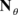are two sets of suitable shape functions written in matrix forms. Introducing the interpolated displacement and rotation fields into the functional of (11) and minimizing the result with respect to nodal values of the variables, the finite element formulation is derived as two sets of equations

 $-\left({\int }_{{\mbox{ }}\Omega }{\left({\mbox{ }}{\mbox{ }}\nabla {\boldsymbol {N}}_{w}\right)}^{T}{\boldsymbol {D}}_{s}{\boldsymbol {N}}_{\theta }{\mbox{ }}{\mbox{ }}d\Omega \right){\mbox{ }}{\mbox{ }}{\mbox{ }}{\overline {\theta }}+$$\left({\int }_{{\mbox{ }}\Omega }{\left({\mbox{ }}{\mbox{ }}\nabla {\boldsymbol {N}}_{w}\right)}^{T}{\boldsymbol {D}}_{s}\nabla {\boldsymbol {N}}_{w}{\mbox{ }}{\mbox{ }}d\Omega \right){\mbox{ }}{\mbox{ }}{\mbox{ }}{\boldsymbol {\overline {w}}}=$${\boldsymbol {f}}_{w}$
(13)

and

 $\left({\int }_{{\mbox{ }}\Omega }{\left({\mbox{ }}{\mbox{ }}{\boldsymbol {L}}{\boldsymbol {N}}_{\theta }\right)}^{T}{\boldsymbol {D}}_{b}{\mbox{ }}{\boldsymbol {L}}{\mbox{ }}{\boldsymbol {N}}_{\theta }{\mbox{ }}{\mbox{ }}d\Omega +\right.$$\left.{\int }_{{\mbox{ }}\Omega }{\boldsymbol {N}}_{\theta }^{T}{\mbox{ }}{\boldsymbol {D}}_{s}{\mbox{ }}{\boldsymbol {N}}_{\theta }{\mbox{ }}{\mbox{ }}d\Omega \right){\mbox{ }}{\mbox{ }}{\mbox{ }}{\overline {\theta }}{\mbox{ }}{\mbox{ }}{\mbox{ }}-$${\mbox{ }}{\mbox{ }}{\mbox{ }}{\mbox{ }}\left({\int }_{{\mbox{ }}\Omega }{\boldsymbol {N}}_{\theta }^{T}{\mbox{ }}{\boldsymbol {D}}_{s}{\mbox{ }}\nabla {\boldsymbol {N}}_{w}{\mbox{ }}{\mbox{ }}d\Omega \right){\mbox{ }}{\mbox{ }}{\mbox{ }}{\boldsymbol {\overline {w}}}=$${\boldsymbol {f}}_{\theta }$
(14)

or in a more compact form

 $\left({\int }_{{\mbox{ }}\Omega }\left[{\mbox{ }}{\mbox{ }}{\boldsymbol {B}}_{b}^{T}{\boldsymbol {D}}_{b}{\mbox{ }}{\boldsymbol {B}}_{b}+\right.\right.$$\left.\left.{\mbox{ }}{\mbox{ }}{\mbox{ }}{\boldsymbol {B}}_{s}^{T}{\boldsymbol {D}}_{s}{\mbox{ }}{\boldsymbol {B}}_{s}\right]{\mbox{ }}{\mbox{ }}d\Omega \right){\mbox{ }}{\mbox{ }}{\mbox{ }}{\boldsymbol {\overline {u}}}=$${\boldsymbol {F}}$
(15)

In which ${\boldsymbol {\overline {u}}}={\left[{\mbox{ }}{\mbox{ }}{\boldsymbol {\overline {w}}}^{T},{\mbox{ }}{\mbox{ }}{\mbox{ }}{\mbox{ }}{\overline {\theta }}^{T}\right]}^{T}$ , ${\boldsymbol {F}}={\left[{\mbox{ }}{\mbox{ }}{\boldsymbol {f}}_{w}^{T},{\mbox{ }}{\mbox{ }}{\mbox{ }}{\boldsymbol {f}}_{\theta }^{T}\right]}^{T}$ , and matrices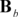and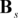are arranged accordingly so that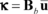, ${\epsilon }_{s}={\boldsymbol {B}}_{s}{\mbox{ }}{\boldsymbol {\overline {u}}}$
(16)

Due to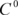nature of the functional, Lagrangian shape functions are usually chosen for the solution. However, such a scheme leads to the so-called “shear locking” effect when thin plates are to be solved. Several remedies have been suggested for overcoming the problem leading to introduction of several kinds of plate elements. In the next section we shall briefly overview those which have been used in this paper.

## 2.2 THE ELEMENTS USED

The locking effect resulting from the application of Reissner-Mindlin formulation to thin plates is overcome by employing special integration schemes or introducing enhanced shear strain fields. Some of the well-adopted kinds of such remedies are explained here.

### 2.2.1 Reduced and selective integration schemes

It is well understood that a quick remedy for locking effect, when using conventional Lagrangian shape-functions, is to use an integration scheme with one order less than the full form. However, if such a scheme is used for all terms in Equation (15), due to some zero energy modes, a sort of instability may happen in the solution process. Therefore selective integration scheme is usually recommended. One of the conventional elements used in this paper is bilinear quadrilateral element for which we shall employ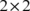and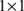Gauss quadrature rules for flexural and shear terms of (15), respectively. Similarly, for quadratic quadrilaterals ( 9 node elements)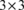integration points for flexural, and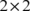integration points for shear terms will be used.

### 2.2.2 Assumed strain formulations

Another remedy for the so called “locking” effect is using assumed variations for shear strains across the elements. Several forms of such elements can be found in literature. Here we shall employ the elements proposed in [21-23]. This class of elements consists of both quadrilateral and triangular elements having similar forms of formulations and has been shown to be robust. The formulations are based on interpolation of shear strains at some sampling points on the edges of the elements. This procedure is called Mixed Interpolation of Tensorial Components, MITC, and the elements are named with the acronym followed by a number associate with the number of nodes in the elements.

MITC4 element

In this type of element the transverse displacement and the two components of the rotation are interpolated by simple linear Lagrangian shape functions and the variations for the shearing strains are assumed as

 ${\gamma }_{xz}=a_{1}+b_{1}{\mbox{ }}\xi$ ${\gamma }_{yz}=a_{2}+b_{2}{\mbox{ }}\eta$

MITC9 element

In this type of element a set of eight-node-based shape functions is used for interpolation of transverse displacement. For rotational components two sets of nine-node-based quadratic shape functions are used. The following forms are assumed for variations of shearing strains

Having found the parameters, the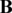matrix in (15) is arranged accordingly.

MITC7 element

In this triangular element the transverse displacement is interpolated by a set of six-node-based shape functions using conventional area coordinates. The two components of rotation are interpolated by two sets of seven-node-based shape functions with the seventh ones, associated with the middle node, being as bubble functions. The variations of shearing strains are assumed as following expressions

 ${\gamma }_{xz}=a_{1}+b_{1}{\mbox{ }}x+c_{1}{\mbox{ }}y+y{\mbox{ }}{\mbox{ }}(d{\mbox{ }}x+$$e{\mbox{ }}y)$ ${\gamma }_{yz}=a_{2}+b_{2}{\mbox{ }}x+c_{2}{\mbox{ }}y+x{\mbox{ }}{\mbox{ }}(d{\mbox{ }}x+$$e{\mbox{ }}y)$

withand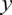being appropriate local orthogonal coordinates. The eight parameters in above expressions are evaluated in terms of shear strains at six sampling points on the edges, located at (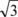/6)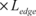from the vertexes, and additional conditions similar to Equation (17).

# 3. THE RECOVERY METHODS

In this paper we shall compare performances of two recovery methods, proven to be superconvergent in two dimensional problems, when applied to plate problems. The superconvergent patch recovery method SPR proposed by Zienkiewicz and Zhu  has been proven to be robust and sufficiently accurate for error estimation. Asymptotic rate of convergence of such an estimate has been studied and compared with other estimates in a series of papers by Babuška and coworkers [3-5]. In this method some sampling points, known to be best at each element, are used and a polynomial is fitted on a group of such points in a patch of elements constructed around a vertex node. Position of the sampling points is known for some simple quadrilateral and triangular elements in two dimensional problems. However, no information is generally available for such points in other kinds of elements.

Another superconvergent recovery method called as Recovery by Equilibrium in Patches REP was introduced in  and shown to have the same accuracy as SPR. This method does not need any information of sampling points. A polynomial is fitted to the gradient values through the equilibrium equations in a manner consistent with the FEM formulation - i.e. the weight form of equilibrium equations. The robustness patch test introduced by Babuška and coworkers [4,5] were applied to the REP and the robustness of the results was compared with those of SPR (see ). It was shown that both methods exhibit the same asymptotic rate of convergence and robustness index.

Here we introduce the general form of the recovery methods for application to plate problems.

## 3.1 THE SUPERCONVERGENT PATCH RECOVERY METHOD

It is well understood that in some simple elements there exist some points where the rate of convergence of the gradients is more than the nominal value of rate in a FEM solution. The locations and other features of such points received a considerable attention in the past two decades. In superconvergent patch recovery method, these points are used as sampling points in a patch of elements constructed on a vertex node. A new continuous distribution of each gradient component is considered as a complete polynomial of order equal to that of the interpolation functions.

For instance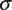, as a representative of the stresses in a FEM solution, is defined as a polynomial with unknown coefficients as

withandbeing two vectors containing the polynomial components and the coefficients, respectively. Summation of the errors between the continuous and discontinuous fields of the stress component is then defined as a norm

and minimized with respect to the unknown coefficients. The result of the minimization is vectoras

The value of the recovered stress at the patch node (the vertex node supporting the patch) is calculated by substitution of (20) in (18) and inserting the node coordinates in the polynomial

The reader is referred to references 6 and 7 for more details. For nodes on the edges of the elements, an interpolation is performed between the recovered values of the stresses from the corresponding continuous fields at the two vertex nodes supporting the edge. The final form of the continuous stress field over the whole domain is constructed by interpolation of the nodal stress values.

For nodes on the boundaries, it is recommended to use the information from the nearest internal patch instead of construction of the patch at the node.

For application of SPR to plate bending problems we shall directly recover the bending moments and shear stress resultants instead of the corresponding stresses by replacing, in Equations (18) to (20), with the components of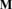andin (7).

For the choice of sampling points in this paper,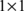andgauss points have been chosen for linear and quadratic quadrilateral elements, respectively. For quadratic triangular elements 3 gauss points, at the middle of the edges, have been used as superconvergent points. It may be noticed that such choices for sampling points are ideal for recovery of the bending moment components since the FEM bending moments are calculated through direct differentiation of the rotation field which is of ${\textstyle C^{0}}$ continuity. This of course corresponds with the original idea of SPR in two dimensional plane stress/strain problems. However, for formulations using enhanced fields of shear stresses/strains, as the case for MITC elements, ambiguities arise in selection of sampling points and thus the need of a separate study is felt in this regard. However, as a reasonable choice for sampling points of shear stresses, we have used the same points as those employed for bending moments because of two reasons. First, it can be observed that information needed for an enhanced field is basically extracted from the derivatives of a ${\textstyle C^{0}}$ field (e.g. see Equation (17)). Note that the final information at the sampling points to be used in the recovery is obtained from the enhanced field. Secondly, using similar sampling points for different stress components speeds up the procedure since the coefficient matrix to be inverted in (20) will be identical for all components.

From above discussion the motive of using another form of recovery method, insensitive to the choice of sampling points, becomes clear. In the next subsection we shall use another recovery which does not exploit information at sampling points.

## 3.2 THE RECOVERY BY EQUILIBRIUM OF PATCHES

The idea of the REP method was inspired from the fact that both finite element stress field and the exact one satisfy a weighted form of the equilibrium equations. This is usually expressed as the following orthogonality condition

 ${\int }_{{\mbox{ }}\Omega }{\boldsymbol {B}}^{T}({\mbox{ }}{\mbox{ }}{\sigma }_{ex}-$${\sigma }_{h}){\mbox{ }}{\mbox{ }}d\Omega ={\boldsymbol {0}}$
(23)

Due to the discontinuity in ${\boldsymbol {B}}$, the condition can be applied, losing no generality, at each vertex node supporting a patch of elements. However, applying such a condition, solely, may not lead to recover any new stress values. If the exact stresses are replaced with some polynomial based stresses then application of (23) to errors of such stress fields, at all nodes in the patch, may lead to construction of the new stress fields. In that case, the orthogonality condition will be one of the applied equations. This leads us to write approximately

 ${\int }_{{\mbox{ }}{\Omega }_{p}}{\boldsymbol {B}}_{p}^{T}{\mbox{ }}{\sigma }^{\ast }d\Omega \approx {\int }_{{\mbox{ }}{\Omega }_{p}}{\boldsymbol {B}}_{p}^{T}{\mbox{ }}{\sigma }_{h}{\mbox{ }}d\Omega$
(24)

It can be seen that the right hand side of Equation (24) is in fact the nodal force vector of the patch and helps the patch to maintain in an equilibrium state. The procedure resembles to the solution of a system, as small as a patch, using a set of piecewise continuous weight functions and a set of generalized coordinates as the polynomial terms. The formulation can be written for strain or even displacement field.

The method was initially proposed in  and then the improved version was given in . In following subsections, we shall extend the formulation for application to plate bending problems.

### 3.2.1 The original form of REP

Writing the equilibrium equations in a weighted from as (24) implies that the new fields of all the stress components should be calculated in a coupled system of equations. Therefore Equation (18) is rewritten in a matrix form

 ${\sigma }^{\ast }={\boldsymbol {P}}{\mbox{ }}{\boldsymbol {\tilde {a}}}$
(25)

Where in plate problems, with five components for stresses, ${\boldsymbol {P}}$ and ${\boldsymbol {\tilde {a}}}$are

Now in view of Equation (15), the equilibrium equations of (24) can be written as:

 ${\int }_{{\mbox{ }}{\Omega }_{p}}{\left[{\boldsymbol {B}}_{b}^{T},{\mbox{ }}{\mbox{ }}{\boldsymbol {B}}_{s}^{T}\right]}_{p}{\boldsymbol {P}}{\mbox{ }}{\boldsymbol {\tilde {a}}}{\mbox{ }}{\mbox{ }}d\Omega \approx {\int }_{{\mbox{ }}{\Omega }_{p}}{\left[{\boldsymbol {B}}_{b}^{T},{\mbox{ }}{\mbox{ }}{\boldsymbol {B}}_{s}^{T}\right]}_{p}\left[{\begin{array}{c}{\boldsymbol {M}}\\{\boldsymbol {S}}\end{array}}\right]{\mbox{ }}{\mbox{ }}{\mbox{ }}d\Omega$
(27)

or

 ${\int }_{{\mbox{ }}{\Omega }_{p}}{\left[{\boldsymbol {B}}_{b}^{T},{\mbox{ }}{\mbox{ }}{\mbox{ }}{\boldsymbol {B}}_{s}^{T}\right]}_{p}{\boldsymbol {P}}{\mbox{ }}{\boldsymbol {\tilde {a}}}{\mbox{ }}{\mbox{ }}d\Omega \approx {\mbox{ }}{\mbox{ }}{\boldsymbol {F}}_{p}$,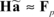(28)

It is clear that for general forms of patches, the number of the equations and the unknowns are not necessarily equal. Thus the following functional is defined

This results to

For some patch configurations, matrix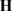may still become ill-conditioned. In such cases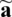can be evaluated as

That is the result of minimization of the following functional

The procedure continues by calculation of the nodal values for stresses and interpolation of these values by the shape functions. This latter part of the method is similar to that of the SPR, stated in the previous subsection, both for inside nodes and those on the boundaries.

### 3.2.2 The improved form of REP

In reference 10 it has been shown that the formulation given for original REP loses robustness for meshes with high aspect ratios. The sensitivity to aspect ratio is eliminated if we write both the finite element and the recovered stress vectors,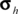and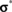, as:

and substitute in Equation (27) to obtain

and split the equilibrium equations which ends up to

In above we have

 ${\boldsymbol {F}}_{i}^{\ast }={\int }_{{\mbox{ }}{\Omega }_{p}}{\boldsymbol {B}}_{p}^{T}{\mbox{ }}{\sigma }_{i}^{\ast }{\mbox{ }}d\Omega$ , ${\boldsymbol {F}}_{i}^{h}={\int }_{{\mbox{ }}{\Omega }_{p}}{\boldsymbol {B}}_{p}^{T}{\mbox{ }}{\sigma }_{h}{}_{i}{\mbox{ }}d\Omega$
(37)

or

 ${\boldsymbol {F}}_{i}^{\ast }={\int }_{{\mbox{ }}{\Omega }_{p}}{\boldsymbol {B}}_{p}^{T}{\boldsymbol {1}}_{i}{\mbox{ }}{\mbox{ }}{\mbox{ }}{\sigma }_{i}^{\ast }d\Omega$ , ${\boldsymbol {F}}_{i}^{h}={\int }_{{\mbox{ }}{\Omega }_{p}}{\boldsymbol {B}}_{p}^{T}{\mbox{ }}{\mbox{ }}{\mbox{ }}{\boldsymbol {1}}_{i}{\sigma }_{h}{}_{i}{\mbox{ }}{\mbox{ }}d\Omega$
(38)

Appropriate columns in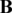matrix may be selected, for nonzero terms only, to minimize the procedure cost.

The split of equilibrium equations, in fact, leads to uncouple the system of equations making the procedure cheaper. Thus for each component of stresses we can write

 ${\int }_{{\mbox{ }}{\Omega }_{p}}{\boldsymbol {B}}_{p}^{T}{\boldsymbol {1}}_{i}{\mbox{ }}{\mbox{ }}{\mbox{ }}({\boldsymbol {p}}^{T}{\boldsymbol {a}}_{i}){\mbox{ }}d\Omega \approx {\boldsymbol {F}}_{i}^{h}$
(39)

or

Now, defining a functional as Equation (29) for each component and minimizing it with respect to the unknowns, the polynomial coefficients for the recovered solution are evaluated

It can be seen that this type of formulation provides more equations when compared to the original form and thus gives better results for robustness tests. This enables us to apply the method to the boundary patches where usually the information for application of SPR is not sufficient. Despite of this, here we shall compare the performances of the two recoveries without using boundary patches.

# 4. ADDING SOME EQUILIBRIUM CONSTRAINTS

Several reports have so far been published on investigation of possible improvement in performance of SPR when augmented with equilibrium constraints. These studies are mainly in two dimensional problems and focus on increasing accuracy and convergence rate of the recovered values. The reader may refer to series of papers written by Wiberg and Abdulwahab [16,17], Blacker and Belytschko  for application of such constraints to plane stress/strain problems. For plate problems, Lee and Hobbs  emphasized on adding equilibrium constraints to the functional (19), similar to the work of Lee et al , to improve the stability of the recovery process. A rather similar constraint, strictly satisfied for the polynomials used, has also been suggested by Okstad et al in . In this paper we shall present a rather comprehensive study on possibility of any improvement in accuracy, stability and rate of convergence of the results when SPR (or REP) is augmented with equilibrium constraints.

## 4.1 EQUILIBRIUM CONSTRAINTS ON SPR

Four forms of constraints are added to the functional used for SPR and the results for each case are distinguished with the acronym followed by a number.

SPR1

In this form we add conditions (9) in square forms to the functional used for SPR

 ${\Pi }_{1}={\Pi }_{0}+w_{1}{\int }_{{\mbox{ }}{\Omega }_{p}}{\left({\mbox{ }}{\boldsymbol {L}}^{T}{\boldsymbol {M}}^{\ast }+{\boldsymbol {S}}^{h}\right)}^{T}\left({\mbox{ }}{\boldsymbol {L}}^{T}{\boldsymbol {M}}^{\ast }+\right.$$\left.{\boldsymbol {S}}^{h}\right){\mbox{ }}{\mbox{ }}d\Omega +$$w_{2}{\int }_{{\mbox{ }}{\Omega }_{\boldsymbol {p}}}({\mbox{ }}{\mbox{ }}{\nabla }^{T}{\boldsymbol {S}}^{\ast }+$$q)^{T}({\mbox{ }}{\mbox{ }}{\nabla }^{T}{\boldsymbol {S}}^{\ast }+$$q){\mbox{ }}{\mbox{ }}d\Omega$
(42)

Where ${\boldsymbol {P}}$ and ${\tilde {\boldsymbol {a}}}$ are defined as (26). In above, the superscriptis used for finite element solutions.

Operators ${\boldsymbol {L}}$ and $\nabla$ are applied to ${\boldsymbol {M}}^{*}$ and ${\boldsymbol {S}}^{*}$ as bellow

 ${\boldsymbol {L}}^{T}{\boldsymbol {M}}^{\ast }=\left[{\begin{array}{ccccc}{\frac {\partial {\boldsymbol {p}}^{T}}{\partial x}}&{\boldsymbol {0}}&{\frac {\partial {\boldsymbol {p}}^{T}}{\partial y}}&{\boldsymbol {0}}&{\boldsymbol {0}}\\{\boldsymbol {0}}&{\frac {\partial {\boldsymbol {p}}^{T}}{\partial y}}&{\frac {\partial {\boldsymbol {p}}^{T}}{\partial x}}&{\boldsymbol {0}}&{\boldsymbol {0}}\end{array}}\right]{\mbox{ }}{\mbox{ }}{\mbox{ }}{\boldsymbol {\tilde {a}}}=$${\boldsymbol {b}}_{b}^{\ast }{\mbox{ }}{\boldsymbol {\tilde {a}}}$
(44)

and

 ${\nabla }^{T}{\boldsymbol {S}}=\left[{\begin{array}{ccccc}{\boldsymbol {0}}&{\boldsymbol {0}}&{\boldsymbol {0}}&{\frac {\partial {\boldsymbol {p}}^{T}}{\partial x}}&{\frac {\partial {\boldsymbol {p}}^{T}}{\partial y}}\end{array}}\right]{\mbox{ }}{\mbox{ }}{\mbox{ }}{\boldsymbol {\tilde {a}}}=$${\boldsymbol {b}}_{s}^{\ast }{\mbox{ }}{\boldsymbol {\tilde {a}}}$
(45)

Now minimization of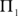with respect to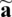leads to the following matrix equation

where

 ${\boldsymbol {\tilde {H}}}=\sum {\boldsymbol {P}}_{i}^{T}{\boldsymbol {P}}_{i}+$$w_{1}{\int }_{{\mbox{ }}{\Omega }_{p}}{\boldsymbol {b}}_{b}^{\ast }{}^{T}{\boldsymbol {b}}_{b}^{\ast }{\mbox{ }}d\Omega +$$w_{2}{\int }_{{\mbox{ }}{\Omega }_{p}}{\boldsymbol {b}}_{s}^{\ast }{}^{T}{\boldsymbol {b}}_{s}^{\ast }{\mbox{ }}d\Omega$
(47)

and

 ${\boldsymbol {\tilde {F}}}=\sum {\boldsymbol {P}}_{i}^{T}{\sigma }_{i}^{h}-$$w_{1}{\int }_{{\mbox{ }}{\Omega }_{p}}{\boldsymbol {b}}_{b}^{\ast }{}^{T}{\boldsymbol {S}}^{h}{\mbox{ }}d\Omega -$$w_{2}{\int }_{{\mbox{ }}{\Omega }_{p}}{\boldsymbol {b}}_{s}^{\ast }{}^{T}q{\mbox{ }}{\mbox{ }}d\Omega$
(48)

SPR2

Instead of requiring shear stress resultants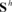to be close to the derivatives of recovered moments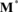, one may apply the equilibrium conditions to the recovered values of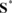and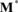both. This simply means that the polynomial coefficients inare interrelated. The following functional is then defined

 ${\Pi }_{2}={\Pi }_{0}+w_{1}{\int }_{{\mbox{ }}{\Omega }_{p}}{\left({\mbox{ }}{\boldsymbol {L}}^{T}{\boldsymbol {M}}^{\ast }+{\boldsymbol {S}}^{\ast }\right)}^{T}\left({\mbox{ }}{\boldsymbol {L}}^{T}{\boldsymbol {M}}^{\ast }+\right.$$\left.{\boldsymbol {S}}^{\ast }\right){\mbox{ }}{\mbox{ }}d\Omega +$$w_{2}{\int }_{{\mbox{ }}{\Omega }_{\boldsymbol {p}}}({\mbox{ }}{\mbox{ }}{\nabla }^{T}{\boldsymbol {S}}^{\ast }+$$q)^{T}({\mbox{ }}{\mbox{ }}{\nabla }^{T}{\boldsymbol {S}}^{\ast }+$$q){\mbox{ }}{\mbox{ }}d\Omega$
(49)

Here we note that

 ${\boldsymbol {L}}^{T}{\boldsymbol {M}}^{\ast }+{\boldsymbol {S}}^{\ast }=$$\left[{\begin{array}{ccccc}{\frac {\partial {\boldsymbol {p}}^{T}}{\partial x}}&{\boldsymbol {0}}&{\frac {\partial {\boldsymbol {p}}^{T}}{\partial y}}&{\boldsymbol {p}}^{T}&{\boldsymbol {0}}\\{\boldsymbol {0}}&{\frac {\partial {\boldsymbol {p}}^{T}}{\partial y}}&{\frac {\partial {\boldsymbol {p}}^{T}}{\partial x}}&{\boldsymbol {0}}&{\boldsymbol {p}}^{T}\end{array}}\right]{\mbox{ }}{\mbox{ }}{\mbox{ }}{\boldsymbol {\tilde {a}}}=$${\boldsymbol {b}}_{bs}^{\ast }{\mbox{ }}{\boldsymbol {\tilde {a}}}$
(50)

Therefore matrices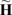and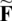in (46), after minimization of the functional, take the form of

 ${\boldsymbol {\tilde {H}}}=\sum {\boldsymbol {P}}_{i}^{T}{\boldsymbol {P}}_{i}+$$w_{1}{\int }_{{\mbox{ }}{\Omega }_{p}}{\boldsymbol {b}}_{bs}^{\ast }{}^{T}{\boldsymbol {b}}_{bs}^{\ast }{\mbox{ }}d\Omega +$$w_{2}{\int }_{{\mbox{ }}{\Omega }_{p}}{\boldsymbol {b}}_{s}^{\ast }{}^{T}{\boldsymbol {b}}_{s}^{\ast }{\mbox{ }}d\Omega$
(51)

and

 ${\boldsymbol {\tilde {F}}}=\sum {\boldsymbol {P}}_{i}^{T}{\sigma }_{i}^{h}-$$w_{2}{\int }_{{\mbox{ }}{\Omega }_{p}}{\boldsymbol {b}}_{s}^{\ast }{}^{T}q{\mbox{ }}{\mbox{ }}d\Omega$
(52)

This latter form of constraints seems to be more reasonable since less information is used from enhanced shear stresses which are not so reliable.

SPR3

Although many authors have used integral forms of equilibrium constraints, a point-wise approach for satisfaction of such conditions may have better interpretation. This is because the exact solution is not necessarily a polynomial but of course may be written as a truncated Taylor series in a tiny area around a point, e.g. patch node. Thus considering the patch node as a point where an enhanced solution is sought, we add to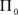a set of point-wise constraints as

 ${\Pi }_{3}={\Pi }_{0}+w_{1}{\left\{{\mbox{ }}{\left({\mbox{ }}{\boldsymbol {L}}^{T}{\boldsymbol {M}}^{\ast }+{\boldsymbol {S}}^{\ast }\right)}^{T}\left({\mbox{ }}{\boldsymbol {L}}^{T}{\boldsymbol {M}}^{\ast }+{\boldsymbol {S}}^{\ast }\right){\mbox{ }}\right\}}_{p-node}+$$w_{2}{\left\{{\mbox{ }}{\left({\mbox{ }}{\mbox{ }}{\nabla }^{T}{\boldsymbol {S}}^{\ast }+q\right)}^{T}({\mbox{ }}{\mbox{ }}{\nabla }^{T}{\boldsymbol {S}}^{\ast }+q){\mbox{ }}\right\}}_{p-node}$
(53)
 ${\boldsymbol {\tilde {H}}}={\sum {\boldsymbol {P}}_{i}^{T}{\boldsymbol {P}}_{i}+w_{1}\left\{{\mbox{ }}{\boldsymbol {b}}_{bs}^{\ast }{}^{T}{\boldsymbol {b}}_{bs}^{\ast }\right\}}_{p-node}+$$w_{2}{\left\{{\mbox{ }}{\boldsymbol {b}}_{s}^{\ast }{}^{T}{\boldsymbol {b}}_{s}^{\ast }\right\}}_{p-node}$
(54)

and

 ${\boldsymbol {\tilde {F}}}=\sum {\boldsymbol {P}}_{i}^{T}{\sigma }_{i}^{h}-$$w_{2}{\left\{{\mbox{ }}{\boldsymbol {b}}_{s}^{\ast }{}^{T}q\right\}}_{p-node}$
(55)

It can be seen that in the case of presence of concentrated load, this form of collocated constraints may not be used since the load intensity,, is not defined. The reader will notice that in the case of existence of a point load on a node, due to the presence of discontinuity in the stress field, the node should be excluded from the nominated ones for patch construction in all forms of stress recovery methods using patches of elements.

SPR4

As the last version of the constraints, we shall use the equilibrium relations between shear stress resultants and bending moments to make links between the recovered shear stress resultants and the derivatives of the bending moments from the standard finite element solution. The motive of using such links is the fact that the FEM results for bending moments are not only more accurate than the shear stress resultants but also are of more convergence rate. Therefore, we examine the following form of constraints

 ${\Pi }_{4}={\Pi }_{0}+w_{1}{\int }_{{\mbox{ }}{\Omega }_{p}}{\left({\mbox{ }}{\boldsymbol {L}}^{T}{\boldsymbol {M}}^{h}+{\boldsymbol {S}}^{\ast }\right)}^{T}\left({\mbox{ }}{\boldsymbol {L}}^{T}{\boldsymbol {M}}^{h}+\right.$$\left.{\boldsymbol {S}}^{\ast }\right){\mbox{ }}{\mbox{ }}d\Omega +$$w_{2}{\int }_{{\mbox{ }}{\Omega }_{\boldsymbol {p}}}({\mbox{ }}{\mbox{ }}{\nabla }^{T}{\boldsymbol {S}}^{\ast }+$$q)^{T}({\mbox{ }}{\mbox{ }}{\nabla }^{T}{\boldsymbol {S}}^{\ast }+$$q){\mbox{ }}{\mbox{ }}d\Omega$
(56)

For evaluation of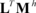one may interpolate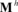through evaluation of the nodal values, by a simple averaging, and compute the first derivatives. Minimization of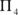with respect toleads to

 ${\boldsymbol {\tilde {H}}}=\sum {\boldsymbol {P}}_{i}^{T}{\boldsymbol {P}}_{i}+$$w_{1}{\int }_{{\mbox{ }}{\Omega }_{p}}{\boldsymbol {p}}_{s}^{\ast }{}^{T}{\boldsymbol {p}}_{s}^{\ast }{\mbox{ }}d\Omega +$$w_{2}{\int }_{{\mbox{ }}{\Omega }_{p}}{\boldsymbol {b}}_{s}^{\ast }{}^{T}{\boldsymbol {b}}_{s}^{\ast }{\mbox{ }}d\Omega$
(57)

and

 ${\boldsymbol {\tilde {F}}}=\sum {\boldsymbol {P}}_{i}^{T}{\sigma }_{i}^{h}-$$w_{1}{\int }_{{\mbox{ }}{\Omega }_{p}}{\boldsymbol {p}}_{s}^{\ast }{}^{T}{\boldsymbol {L}}^{T}{\boldsymbol {M}}^{h}d\Omega -$$w_{2}{\int }_{{\mbox{ }}{\Omega }_{p}}{\boldsymbol {b}}_{s}^{\ast }{}^{T}q{\mbox{ }}{\mbox{ }}d\Omega$
(58)

Where

In the section of numerical results, we shall give a study on accuracy of the recovered stresses when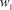and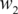take different values.

## 4.2 CONSTRAINTS ON REP, (REP1)

Although REP procedure is in fact application of a weighted form of equilibrium operator to the recovered stress fields, we shall add only a set of constraints, on its original form, as Equation (42) withreplaced with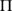in (29). The derivation of the relations is omitted here.

# 5. NUMERICAL STUDY

To study the performances of the recovery methods, two main approaches available in literature may be employed. The first approach uses benchmark problems with exact solutions to demonstrate the accuracy of the recovered solutions. The idea supporting such an approach is to generalize the conclusions to wider range of problems though no proof is given. Majority of studies on error estimators fall within this form of approach [2,6,7,9,15-18,24,27,29].

In the second approach, asymptotic behaviors of the error estimators are studied. Although such a study does not play the role of a proof, but the mathematical basis is much stronger and the range of application is much wider than the first approach. Pioneering works by Babuška and coworkers for two-dimensional heat and plane stress/strain problems fall within this form of approach [3-5]. The methodology presented in these studies was later used to test the performance of REP in reference 10.

For plate bending problems, however, application of the second approach requires generalization of the methodology for mixed solution methods such as Reissner-Mindlin formulation, which is beyond the scopes of this paper. The reader may consult to references 19 and 20 for the mathematical model and benchmark examples performed for linear rectangular elements.

While using the first approach, problems with smooth exact solutions are needed. Here the term “smooth” indicates that no singularity is expected in the exact stress field. Searching for benchmarks in plate bending problems with perfect smooth exact solutions is not an easy task. In fact in many problems, especially in thin plates, boundary layer effect induced by shear forces at the edges of the plate plays the role of singularity. But, since such a boundary layer is just seen in transverse shear stresses and has small effect on bending stresses, it is neglected when the plates are designed for bending stresses.

Figure 1. A simply supported square; ${\frac {t}{L}}=0.2$ for thick plate solution and ${\frac {t}{L}}=0.002$ for thin plate solution (a) the domain (b) typical uniform meshes of quadrilateral, solid lines, and triangular, dash lines, used.

In this paper a square plate, shown in Figure 1, with hard simply supports under uniform pressure is considered as a benchmark problem. Depending on the thickness of the plate, the exact solution is given with assumption of thick or thin plate formulation. Fourier series is usually used for both cases expressing the plate deflection as follows

withandbeing the plate dimensions and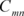representing a series of coefficients to be determined from the solution process. For thin plate solution, the deflection assumed above is directly substituted in to the governing differential equation derived from Kirchhoff assumptions. The solution is straightforward. For thick plate solution, however, some similar expressions for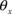and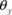are needed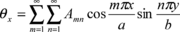, ${\theta }_{y}=\sum _{m=1}^{\infty }\sum _{n=1}^{\infty }B_{mn}sin{\frac {m\pi x}{a}}cos{\frac {n\pi y}{b}}$
(61)

The solution may be found in reference 34.

In this section we shall present two series of results obtained for the thick and thin plates. The first series is of comparison between the performances of the two recovery methods SPR and REP. The second series will be given to demonstrate the performances of the recovery methods when the equilibrium constraints in Section 4 are applied.

The comparisons will be made by evaluation of relative norms of errors. Some norms used in this section are as follows

 Total energy norm: ${\Vert {\boldsymbol {u}}\Vert }_{t}={\left({\Vert {\boldsymbol {u}}\Vert }_{b}^{2}+{\Vert {\boldsymbol {u}}\Vert }_{s}^{2}\right)}^{\frac {1}{2}}$
(62)
 Energy norm of bending stresses: ${\Vert {\boldsymbol {u}}\Vert }_{b}={\left({\int }_{{\mbox{ }}\Omega }{\boldsymbol {M}}^{T}{\boldsymbol {D}}_{b}^{-1}{\boldsymbol {M}}{\mbox{ }}{\mbox{ }}d\Omega \right)}^{\frac {1}{2}}$
(63)
 Energy norm of shear stresses: ${\Vert {\boldsymbol {u}}\Vert }_{s}={\left({\int }_{{\mbox{ }}\Omega }{\boldsymbol {S}}^{T}{\boldsymbol {D}}_{s}^{-1}{\boldsymbol {S}}{\mbox{ }}{\mbox{ }}{\mbox{ }}d\Omega \right)}^{\frac {1}{2}}$
(64)

For recovered values we define

 Energy norm of errors: ${\Vert {\boldsymbol {e}}\Vert }_{t}={\left({\Vert {\boldsymbol {e}}\Vert }_{b}^{2}+{\Vert {\boldsymbol {e}}\Vert }_{s}^{2}\right)}^{\frac {1}{2}}$
(65)
 Energy norm of errors for bending stresses: ${\Vert {\boldsymbol {e}}\Vert }_{b}={\left({\int }_{{\mbox{ }}\Omega }{\left({\boldsymbol {M}}-{\boldsymbol {M}}^{\ast }\right)}^{T}{\boldsymbol {D}}_{b}^{-1}({\boldsymbol {M}}-{\boldsymbol {M}}^{\ast }){\mbox{ }}{\mbox{ }}d\Omega \right)}^{\frac {1}{2}}$
(66)
 Energy norm of errors for shear stresses: ${\Vert {\boldsymbol {e}}\Vert }_{s}={\left({\int }_{{\mbox{ }}\Omega }{\left({\boldsymbol {S}}-{\boldsymbol {S}}^{\ast }\right)}^{T}{\boldsymbol {D}}_{s}^{-1}({\boldsymbol {S}}-{\boldsymbol {S}}^{\ast }){\mbox{ }}{\mbox{ }}d\Omega \right)}^{\frac {1}{2}}$
(67)

Thus the associative relative error norms are defined as

 ${\eta }_{t}={\frac {{\Vert {\boldsymbol {e}}\Vert }_{t}}{{\Vert {\boldsymbol {u}}\Vert }_{t}}}\times 100$ , ${\eta }_{b}={\frac {{\Vert {\boldsymbol {e}}\Vert }_{b}}{{\Vert {\boldsymbol {u}}\Vert }_{b}}}\times 100$ , ${\eta }_{s}={\frac {{\Vert {\boldsymbol {e}}\Vert }_{s}}{{\Vert {\boldsymbol {u}}\Vert }_{s}}}\times 100$
(68)

The finite element solutions are obtained on uniform meshes of square elements and triangular elements with regular pattern.

## 5.1 Comparison between SPR and REP

Thick plate solution

The plate shown in Figure 1 has been solved with ${\frac {t}{L}}=0.2$ as a case of thick plate problems. The results of errors and the rates of convergence are reported in Tables 2-12 and Figures 2-4.

Table 2. Point-wise errors (percent) of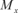at point A, MITC4.

 h FEM SPR REP-old REP 2.0 1.0 0.5 0.333 0.222 C.R. 45.60 14.11 5.71 3.62 2.34 1.351 28.10 4.72 1.69 0.75 0.33 2.018 23.72 3.83 1.38 0.61 0.27 2.034 26.97 4.48 1.60 0.71 0.32 2.025

Table 3. Point-wise errors (percent) of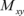at point A, MITC4.

 h FEM SPR REP-old REP 2.0 1.0 0.5 0.333 0.222 C.R. 37.18 21.97 10.63 7.14 4.78 0.933 17.18 4.68 0.87 0.38 0.17 2.015 6.37 1.13 0.26 0.11 0.05 2.207 16.46 4.44 0.83 0.37 0.16 2.107

Table 4. Point-wise errors (percent) of $S_{x}$ at point A, MITC4.

 h FEM SPR REP-old REP 2.0 1.0 0.5 0.333 0.222 C.R. 86.00 35.62 16.26 10.61 6.98 1.143 30.76 4.45 0.34 0.15 0.07 2.791 10.54 1.93 0.22 0.11 0.05 2.398 46.44 3.73 0.21 0.09 0.04 3.191

Table 5. Point-wise errors (percent) ofat point A, MITC7.

 h SPR REP-old REP 2.0 1.0 0.666 C.R. 1.942 0.102 0.019 4.216 8.872 2.587 1.351 1.713 1.437 0.06 0.02 3.882

Table 6. Point-wise errors (percent) ofat point A, MITC7.

 h SPR REP-old REP 2.0 1.0 0.666 C.R. 1.042 0.162 0.033 3.154 1.757 2.277 1.403 --- 1.169 0.084 0.010 4.325

Table 7. Point-wise errors (percent) of $S_{x}$ at point A, MITC7.

 h SPR REP-old REP 2.0 1.0 0.666 C.R. 1.600 0.066 0.013 4.367 1.821 3.394 5.515 4.508 0.429 0.084 3.625

Table 8. Relative error norms for thick plate solution using MITC9 elements.

 FEM SPR REP-old REP h Bend. Shear Total ${\textstyle {\eta }_{b}}$ ${\textstyle {\eta }_{s}}$ ${\textstyle {\eta }_{t}}$ Bend. Shear Total ${\textstyle {\eta }_{b}}$ ${\textstyle {\eta }_{s}}$ ${\textstyle {\eta }_{t}}$ Bend. Shear Total ${\textstyle {\eta }_{b}}$ ${\textstyle {\eta }_{s}}$ ${\textstyle {\eta }_{t}}$ Bend. Shear Total ${\textstyle {\eta }_{b}}$ ${\textstyle {\eta }_{s}}$ ${\textstyle {\eta }_{t}}$ 2.00 1.33 1.00 .667 .533 .400 C.R. 8.12 5.02 7.63 3.55 2.12 3.32 1.90 1.16 1.79 0.77 0.52 0.73 0.47 0.34 0.45 0.24 0.21 0.75 2.180 1.981 2.157 4.53 5.54 4.74 1.42 2.29 1.63 0.61 1.26 0.78 0.18 0.56 0.29 0.10 0.36 0.18 0.004 0.21 0.10 2.938 2.036 2.406 8.58 10.33 8.94 2.63 3.79 2.89 1.16 1.97 1.35 0.37 0.84 0.49 0.20 0.53 0.29 0.009 0.31 0.16 2.803 2.182 2.647 6.76 5.23 6.49 2.23 2.08 2.20 0.99 1.77 1.03 0.32 0.52 0.36 0.53 0.34 0.21 0.007 0.20 0.11 2.809 2.041 2.544

Table 9. Point-wise errors (percent) ofat point A, MITC9.

 h FEM SPR REP-old REP 2.0 1.0 0.666 0.400 C.R. 10.59 3.73 1.33 0.375 2.075 0.815 0.078 0.016 0.002 3.707 1.884 0.078 0.010 0.0006 5.007 1.036 0.180 0.044 0.0079 3.032

Table 10. Point-wise errors (percent) ofat point A, MITC9.

 h FEM SPR REP-old REP 2.0 1.0 0.666 0.400 C.R. 16.38 2.08 0.49 0.046 3.644 5.31 0.206 0.029 0.003 4.617 16.47 0.395 0.062 0.008 4.705 5.51 0.253 0.049 0.005 4.299

Table 11. Point-wise errors (percent) of $S_{x}$ at point A, MITC9.

 h FEM SPR REP-old REP 2.0 1.0 0.666 0.400 C.R. 29.19 2.91 0.81 0.28 2.878 2.713 0.106 0.014 0.002 4.489 10.91 0.895 0.289 0.044 3.420 3.32 0.101 0.010 0.002 4.648

Table 12. Relative error norms for thick plate solution using conventional 9 node elements.

 FEM SPR REP-old REP h Bend. Shear Total ${\textstyle {\eta }_{b}}$ ${\textstyle {\eta }_{s}}$ ${\textstyle {\eta }_{t}}$ Bend. Shear Total ${\textstyle {\eta }_{b}}$ ${\textstyle {\eta }_{s}}$ ${\textstyle {\eta }_{t}}$ Bend. Shear Total ${\textstyle {\eta }_{b}}$ ${\textstyle {\eta }_{s}}$ ${\textstyle {\eta }_{t}}$ Bend. Shear Total ${\textstyle {\eta }_{b}}$ ${\textstyle {\eta }_{s}}$ ${\textstyle {\eta }_{t}}$ 2.00 1.33 1.00 .667 .533 .400 C.R. 3.21 7.85 4.48 1.48 3.69 2.09 0.85 2.14 1.21 0.38 0.97 0.54 0.25 0.62 0.35 0.14 0.35 0.20 1.955 1.938 1.945 3.96 5.14 4.21 1.28 2.17 1.49 0.56 1.20 0.73 0.17 0.54 0.28 0.09 0.35 0.17 0.04 0.20 0.09 2.896 2.011 2.360 8.26 8.22 8.25 2.58 3.04 2.68 1.12 1.60 1.23 0.34 0.71 0.44 0.18 0.46 0.25 0.07 0.26 0.13 2.923 2.150 2.592 6.15 4.97 5.94 2.06 2.10 2.07 0.92 1.21 0.98 0.29 0.55 0.35 0.15 0.36 0.21 0.06 0.21 0.10 2.829 1.974 2.532

Figure 2 demonstrates the performances of SPR and REP when MITC4 elements are used. As expected, the average rate of convergence for FEM solution is close to unity while those of the recovery methods are more meaning that the recovery methods are superconvergent similar to simple two-dimensional problems. It can be seen that the convergence rates for the recovery methods are similar, though small differences are observed. Point-wise errors at point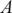in Figure 1 are given in Tables 2 to 4. Nearly the same rates of convergence as energy norms are observed indicating that all points in the domain have similar rates of convergence.

Figure 2. Relative error norms for thick plate solution using MITC4 elements; (a) error for bending stresses ${\textstyle {\eta }_{b}}$ , (b) error for shear stresses ${\textstyle {\eta }_{s}}$ , (c) total error ${\textstyle {\eta }_{t}}$ .

Similar conclusions may be made from the results of Figure 3 where conventional linear elements are used.

Rather surprising results can be seen in Figure 4 where the thick plate solution has been obtained using MITC7 elements. None of the recovery methods appear to be fully superconvergent in terms of energy norms, though they exhibit more convergent rates when compared to FEM solution, especially for bending stresses. It can be seen that REP-old, due to instability, fails to be superconvergent but the improved version of REP retains to be semi-superconvergent, similar to SPR. However, looking at Tables 5 to 7 for point-wise errors at, it can be concluded that the main reason for semi-superconvergent behavior lies in the treatment of boundary patches since the point-wise results show quite good superconvergent (or ultraconvergent) effects except for REP-old. In this paper, the recovered gradients at boundary nodes, for both SPR and two versions of REP, are obtained through extrapolation of information from closest inside patch. We note that, although in the improved form of REP there is no restriction for applying the formulation to a local boundary patch, no boundary patch has been used in this paper in order to compare REP and SPR in similar situations.

Figure 3. Relative error norms for thick plate solution using conventional 4 node elements; (a) error for bending stresses ${\textstyle {\eta }_{b}}$ , (b) error for shear stresses ${\textstyle {\eta }_{s}}$ , (c) total error ${\textstyle {\eta }_{t}}$ .

Figure 4. Relative error norms for thick plate solution using MITC7 elements; (a) error for bending stresses ${\textstyle {\eta }_{b}}$ , (b) error for shear stresses ${\textstyle {\eta }_{s}}$ , (c) total error ${\textstyle {\eta }_{t}}$ .

A similar conclusion can be made for quadratic elements MITC9. Table 8 demonstrates the performances of the recovery methods for this class of elements. Here again, when using energy norms, superconvergence is lost in recovered shear stresses, though the recovered bending stresses still exhibit better behavior. Similar conclusions for quadratic triangular elements are also valid here since the point-wise errors in Tables 9 to 11 demonstrate good superconvergent/ultraconvergent effects.

The results for conventional nine node elements, shown in Table 12, exhibit nearly the same behavior as MITC9 elements.

Thin plate solution

The plate shown in Figure 1 has been solved with ${\frac {t}{L}}=0.002$ as a case of thin plate problems. The results of errors and the rates of convergence are reported in Tables 13 and 14 and Figures 5 and 6.

The summery of the results for a set of solutions using MITC4 elements is reported in Figure 5. Similar results have been obtained for the recovery methods. Both shear and bending stresses norms exhibit superconvergent effect. The reader may note that the errors in the figure are relative quantities and do not clearly show the contribution of each part of the energy in the total energy error. However, similarity between Figures (5-a) and (5-c) reveals that the contribution of shear part is very small. In thin plate formulation, the bending stresses have a prominent role in the plate behavior and thus the shear stresses are of less importance.

Figure 5. Relative error norms for thin plate solution using MITC4 elements; (a) error for bending stresses ${\textstyle {\eta }_{b}}$ , (b) error for shear stresses ${\textstyle {\eta }_{s}}$ , (c) total error ${\textstyle {\eta }_{t}}$ .

Table 13 shows results obtained when MITC7 elements are used. Rather good results have been obtained for bending stress norms. The errors for shear stress norms from FEM solution show that the shear stress field obtained is not so accurate and when the element size decreases the convergence rate expected for FEM solution is not retrieved. It is worthwhile to note that the element was invented to solve the locking effect in thin plate formulation and thus the accuracy of the shear stresses is of no importance. However, it can be seen that both SPR and REP are able to recover the shear stress field with reasonable convergence rate, though the original form of REP fails to produce convergent results.

Table 13. Relative error norms for thin plate solution using MITC7 elements.

 FEM SPR REP-old REP h Bend. Shear Total ${\textstyle {\eta }_{b}}$ ${\textstyle {\eta }_{s}}$ ${\textstyle {\eta }_{t}}$ Bend. Shear Total ${\textstyle {\eta }_{b}}$ ${\textstyle {\eta }_{s}}$ ${\textstyle {\eta }_{t}}$ Bend. Shear Total ${\textstyle {\eta }_{b}}$ ${\textstyle {\eta }_{s}}$ ${\textstyle {\eta }_{t}}$ Bend. Shear Total ${\textstyle {\eta }_{b}}$ ${\textstyle {\eta }_{s}}$ ${\textstyle {\eta }_{t}}$ 2.00 1.33 1.00 .667 .533 C.R. 29.25 26.40 29.25 18.04 25.63 18.04 13.11 25.97 13.11 8.51 23.62 8.51 6.75 22.77 6.75 1.109 0.112 1.489 14.29 16.28 14.29 6.97 12.31 6.98 4.22 10.48 4.23 2.30 7.96 2.10 1.43 6.63 1.43 1.741 0.679 1.740 30.83 27.79 30.83 29.21 36.51 29.22 25.06 53.50 25.07 24.08 81.12 24.09 21.69 88.10 21.69 0.266 - 0.266 29.84 17.18 29.84 14.46 12.98 14.46 8.85 10.92 8.85 4.24 8.39 4.24 2.83 7.10 2.83 1.781 0.668 1.735

Performance of quadratic quadrilateral plate elements MITC9 has been shown in Figure 6. It can be seen that, similar to thick plate solution cases, all recovery methods produce semi-superconvergent results.

Figure 6. Relative error norms for thin plate solution using MITC9 elements; (a) error for bending stresses ${\textstyle {\eta }_{b}}$ , (b) error for shear stresses ${\textstyle {\eta }_{s}}$ , (c) total error ${\textstyle {\eta }_{t}}$ .

Convergence rate obtained for stress resultants at point A , for all above cases, is reported in Table 14. Among the results for different types of elements in this table, those of MITC9 are notable which show ultraconvergence rates. Similar convergences were seen in Tables 9 to 11 for thick plate solution. Ultraconvergence of SPR was first reported by Zienkiewicz and Zhu in reference 6 in two dimensional plane stress/strain problems. The effect was later proven by Zhang  for quadrilateral elements. Also, ultraconvergence of REP was shown in reference 9 for two dimensional problems.

Table 14. Rate of convergence of stresses at point A - Thin plate solution using different types of elements.

 Stress resultant Recovery method MITC4 elements MITC7 elements MITC9 elementsSPR 2.019 1.089 3.733 REP-old 2.038 2.604 5.253 REP 2.026 1.092 2.875SPR 2.149 0.929 4.768 REP-old 2.395 0.866 4.727 REP 2.154 0.928 3.908 $S_{x}$ SPR 1.882 1.881 3.742 REP-old 1.489 ---- 3.151 REP 1.962 1.791 2.997

## 5.2 Application of the equilibrium constraints

In this part we shall investigate the possibility of any improvement in the accuracy or the rate of convergence of the results when the constraints described in Section 4 are applied.

To proceed towards our investigation, we need to choose proper values for the weights used for the constraints in the functionals (42), (49), (53) and (56). This, in fact, needs a separate study which we shall present the results prior to study on the rates of convergence.

Figures 7 depicts the variation of relative errors, to be interpreted as the accuracy in a thick plate solution, with respect to variation of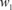and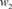for SPR1 type of constraints when a mesh of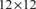quadratic elements (MITC9) is used. The figures clearly show that no major improvement happens when the constraints are applied. Although an optimal value can be recognized for, the solution is not much sensitive to the values of the weights.

Figure 7. Variation of relative errors with respect to variation of weight factors, ${\textstyle w_{1}}$ and ${\textstyle w_{2}}$ , for thick plate solution using MITC9 elements and when SPR1 recovery is applied; (a) variation of error norm for bending stresses ${\textstyle {\eta }_{b}}$ , (b) variation of error norm for shear stresses ${\textstyle {\eta }_{s}}$ .

For SPR2 type of constraints, Figure 8 shows that the solution is only sensitive tobut the accuracy deteriorates whileincreases. This means that enforcing the recovered shear and bending stresses to be interrelated, in an integral form, will not lead to better results (viz. functional (49)). The optimal values, though not recognizable from the figures, are less than unity. Here again, no major improvement can be seen in the figures.

Figure 8. Variation of relative errors with respect to variation of weight factors, ${\textstyle w_{1}}$ and ${\textstyle w_{2}}$ , for thick plate solution using MITC9 elements and when SPR2 recovery is applied; (a) variation of error norm for bending stresses ${\textstyle {\eta }_{b}}$ , (b) variation of error norm for shear stresses ${\textstyle {\eta }_{s}}$ .

Figure 9 depicts the results for SPR3 type of formulation where point-wise forms of constraints have been used. Although, here again, the improvement is not significant, optimal values can be found forand. This means that adding point-wise constraints may lead to slightly better results.

Figure 9. Variation of relative errors with respect to variation of weight factors, ${\textstyle w_{1}}$ and ${\textstyle w_{2}}$ , for thick plate solution using MITC9 elements and when SPR3 recovery is applied; (a) variation of error norm for bending stresses ${\textstyle {\eta }_{b}}$ , (b) variation of error norm for shear stresses ${\textstyle {\eta }_{s}}$ .

For SPR4, just the shear energy norm has been selected to demonstrate the results since in functional (56) the constraints have been applied to recovered shear stresses only. Figure 10 shows that relating the recovered shear stresses to derivatives of bending moment does not improve the results. Similar conclusion is made from Figure 11 for constraints on REP1.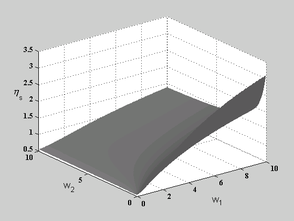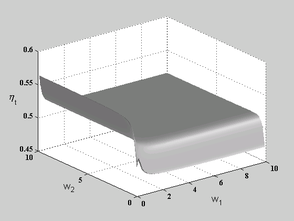Figure 10. Variation of error norm for shear stresses (${\textstyle {\eta }_{s}}$) with respect to variation of weight factors, ${\textstyle w_{1}}$ and ${\textstyle w_{2}}$ , for thick plate solution using MITC9 elements and when SPR4 recovery is applied. Figure 11. Variation of energy norm of error (${\textstyle {\eta }_{t}}$) with respect to variation of weight factors, ${\textstyle w_{1}}$ and ${\textstyle w_{2}}$ , for thick plate solution using MITC9 elements and when REP1 recovery is applied.

So far we have shown that adding equilibrium constraints to original form of SPR does not much improve the accuracy of the solution. This implicitly means that the recovered fields of stresses, obtained from the original form, are very close to equilibrium state.

Now, we are ready to examine the effect of constraints on the convergence rate of the recovered solution. For each case we have chosen the optimal values forandfrom the previous study. The results for the energy norms are reported in Tables 15 to 18 for thin and thick plate solutions. To perform the study we compare the rates in Figure 2, for original form of SPR, with those of Table 15 for thick plate solution using MITC4 elements. No significant change can be seen in the convergence rates. Among the four forms of SPR the second one, SPR2, shows a slight increase in the shear energy norm rate.

Table 15. Relative error norms for thick plate solution using MITC4 elements. A comparison between various forms of constraints on SPR.

 SPR1 SPR2 SPR3 SPR4 h Bend. Shear Total ${\textstyle {\eta }_{b}}$ ${\textstyle {\eta }_{s}}$ ${\textstyle {\eta }_{t}}$ Bend. Shear Total ${\textstyle {\eta }_{b}}$ ${\textstyle {\eta }_{s}}$ ${\textstyle {\eta }_{t}}$ Bend. Shear Total ${\textstyle {\eta }_{b}}$ ${\textstyle {\eta }_{s}}$ ${\textstyle {\eta }_{t}}$ Bend. Shear Total ${\textstyle {\eta }_{b}}$ ${\textstyle {\eta }_{s}}$ ${\textstyle {\eta }_{t}}$ 2.00 1.00 .667 .500 .333 .222 C.R. 26.79 18.99 25.48 8.67 4.97 8.35 3.96 2.37 3.71 2.34 1.36 2.19 1.10 0.63 1.02 0.51 0.30 0.47 1.806 1.886 1.814 26.80 37.78 29.23 8.60 9.51 8.93 3.96 3.76 3.92 2.34 1.92 2.26 1.10 0.78 1.04 0.51 0.34 0.48 1.807 2.147 1.873 26.80 19.34 25.54 8.53 5.06 8.34 3.96 2.40 3.72 2.34 1.38 2.19 1.10 0.64 1.03 0.51 0.31 0.47 1.807 1.888 1.814 26.71 19.12 25.43 8.87 4.99 8.55 3.96 2.38 3.71 2.34 1.37 2.19 1.10 0.64 1.02 0.51 0.30 0.47 1.805 1.887 1.813

Table 16. Relative error norms for thick plate solution using MITC9 elements. A comparison between various forms of constraints on SPR.

 SPR1 SPR2 SPR3 SPR4 h Bend. Shear Total ${\textstyle {\eta }_{b}}$ ${\textstyle {\eta }_{s}}$ ${\textstyle {\eta }_{t}}$ Bend. Shear Total ${\textstyle {\eta }_{b}}$ ${\textstyle {\eta }_{s}}$ ${\textstyle {\eta }_{t}}$ Bend. Shear Total ${\textstyle {\eta }_{b}}$ ${\textstyle {\eta }_{s}}$ ${\textstyle {\eta }_{t}}$ Bend. Shear Total ${\textstyle {\eta }_{b}}$ ${\textstyle {\eta }_{s}}$ ${\textstyle {\eta }_{t}}$ 2.00 1.33 1.00 .667 .533 .400 C.R. 4.27 5.46 4.52 1.34 2.23 1.56 0.58 1.23 0.75 0.18 0.54 0.28 0.09 0.35 0.17 0.04 0.20 0.09 2.922 2.050 2.404 4.15 7.89 5.08 1.31 2.77 1.69 0.56 1.40 0.80 0.17 0.58 0.30 0.09 0.37 0.18 0.04 0.21 0.10 2.926 2.245 2.446 4.55 5.63 4.77 1.43 2.33 1.64 0.61 1.28 0.79 0.19 0.56 0.30 0.10 0.36 0.18 0.04 0.21 010 2.922 2.044 2.411 4.53 5.47 4.72 1.42 2.25 1.62 0.61 1.24 0.77 0.18 0.55 0.29 0.10 0.35 0.18 0.04 0.20 0.10 2.938 2.043 2.417

Table 17. Relative error norms for thin plate solution using MITC4 elements. A comparison between various forms of constraints on SPR.

 SPR1 SPR2 SPR3 SPR4 h Bend. Shear Total ${\textstyle {\eta }_{b}}$ ${\textstyle {\eta }_{s}}$ ${\textstyle {\eta }_{t}}$ Bend. Shear Total ${\textstyle {\eta }_{b}}$ ${\textstyle {\eta }_{s}}$ ${\textstyle {\eta }_{t}}$ Bend. Shear Total ${\textstyle {\eta }_{b}}$ ${\textstyle {\eta }_{s}}$ ${\textstyle {\eta }_{t}}$ Bend. Shear Total ${\textstyle {\eta }_{b}}$ ${\textstyle {\eta }_{s}}$ ${\textstyle {\eta }_{t}}$ 2.00 1.00 .667 .500 .333 .222 C.R. 26.80 18.73 25.80 8.25 4.66 8.24 3.95 2.35 3.95 2.33 1.36 2.33 1.09 0.64 1.09 0.50 0.31 0.50 1.808 1.874 1.808 26.81 37.67 26.81 8.31 8.46 8.31 3.95 3.72 3.93 2.33 1.90 2.33 1.09 0.78 1.09 0.50 0.34 1.60 1.808 2.142 1.808 26.80 19.07 26.80 8.38 4.87 8.38 3.96 2.39 3.96 2.34 1.38 2.34 1.09 0.65 1.09 0.50 0.31 0.50 1.901 1.875 1.808 26.73 19.11 26.73 8.15 4.81 8.15 3.95 2.37 3.95 2.33 1.37 2.33 1.09 0.64 1.09 0.50 0.31 0.50 1.807 1.874 1.807

Table 18. Relative error norms for thin plate solution using MITC9 elements. A comparison between various forms of constraints on SPR.

 SPR1 SPR2 SPR3 SPR4 h Bend. Shear Total ${\textstyle {\eta }_{b}}$ ${\textstyle {\eta }_{s}}$ ${\textstyle {\eta }_{t}}$ Bend. Shear Total ${\textstyle {\eta }_{b}}$ ${\textstyle {\eta }_{s}}$ ${\textstyle {\eta }_{t}}$ Bend. Shear Total ${\textstyle {\eta }_{b}}$ ${\textstyle {\eta }_{s}}$ ${\textstyle {\eta }_{t}}$ Bend. Shear Total ${\textstyle {\eta }_{b}}$ ${\textstyle {\eta }_{s}}$ ${\textstyle {\eta }_{t}}$ 2.00 1.33 1.00 .667 .533 .400 C.R. 4.34 6.15 4.34 1.38 2.64 1.38 0.60 1.50 0.60 0.18 0.69 0.18 0.09 0.44 0.09 0.04 0.25 0.04 2.912 1.981 1.904 4.24 8.45 4.24 1.34 3.13 1.34 0.58 1.65 0.58 0.18 0.71 0.18 0.09 0.45 0.09 0.04 0.26 0.04 2.918 2.163 2.910 4.57 6.15 4.57 1.47 2.64 1.47 0.64 1.49 0.64 0.19 0.68 0.19 0.10 0.44 0.10 0.04 0.25 0.04 2.891 1.985 2.885 4.54 6.08 4.54 1.45 2.61 1.45 0.63 1.48 0.63 0.19 0.68 0.19 0.10 0.44 0.10 0.04 0.25 0.04 2.905 1.980 2.897

The same conclusion can be made for thick and thin plate solutions, reported in Tables 16 to 18, when they are compared to those of Tables 8, Figures 5 and 6, respectively.

It can clearly be seen that adding some equilibrium constraints, has almost no effect on the results of SPR for both accuracy and the rate of convergence. Here we have not reported the results for triangular elements since the same conclusion is also made for this class of elements.

To complete our studies, we report the results of a constrained form of REP as Table 19 (see subsection 4.2). Here the original form of REP has been used since the formulation is written in a couple form and thus additional constraints does not increase the computational cost siginificantly. Since REP formulation is, in fact, based on a weighted form of equilibrium, as expected, no improvement is seen in the results of Table.

Table 19. Relative error norms for thick plate solution using MITC9 elements. Performance of REP1 type of formulation.
 h Bend. Shear Total ${\textstyle {\eta }_{b}}$ ${\textstyle {\eta }_{s}}$ ${\textstyle {\eta }_{t}}$ 2.00 1.33 1.00 .667 .533 .400 C.R. 7.60 9.09 7.90 2.44 3.33 2.64 1.11 1.76 1.26 0.37 0.79 0.48 0.21 0.52 0.29 0.10 0.31 0.02 2.710 2.106 2.423

# 6. CONCLUSIONS

In this paper the formulation of two recovery methods, proven to be superconvergent in two dimensional problems i.e. SPR and REP, have been extended and applied to plate problem using Reissner-Mindlin type of formulation. The elements used are basically those which have been recently proposed in [21-23] for eliminating “locking” effect, namely the series of MITC elements. The study has been performed on a benchmark problem used for both thick and thin cases.

Comparison of the results for SPR and REP shows that the improved version of REP gives similar results to those of SPR, though it does not require superconvergent sampling points. The original form of REP sometimes fails to give convergent results, however, in some point wise results it shows ultraconvergent rates.

For MITC4 elements, both SPR and REP, show nearly full superconvergent behavior for energy norms as well as point-wise values.

For quadratic elements MITC7 and MITC9, however, SPR and REP show semi-superconvergent behavior for energy norms but much better convergence rates are seen for point-wise values. This implies that, for these types of elements, the recovery procedures are very sensitive to the treatment of the boundary patches. In this paper for recovered values at boundaries we have extrapolated the values obtained from the closest interior patches for both SPR and REP.

The results obtained for thick and thin plate solutions basically exhibit similar rate of convergence and the accuracy for bending stresses. For shear stresses, however, the results of thick plate solution show superconvergent effect though those of thin plate solution, especially for MITC7 do not show the desired effects. We notice that, for this type of elements, although the finite element solution is poor regarding accuracy and convergence rate, the element can successfully eliminate the “locking” effect. Moreover, we note that the shearing part of the energy in thin plate solution, compared to bending part, is very small and has little contribution in an adaptive procedure. This means that this element, also, can effectively be used in adaptive procedures for thick and thick plate solutions, using recovery based estimates.

In another attempt we have investigated the effects of some equilibrium constraints on the results of SPR procedure. The constraints have been designed in integral and point-wise forms. The study consists of two parts. First the improvement in the accuracy of the recovered field has been examined and then a study has been performed on the effects of the constraints on the convergence rate. No significant improvement can be seen in the results regarding both accuracy and the convergence rate. This implies that the application of the uncoupled form of SPR leads to best results.

In this paper we have not applied boundary constraints to the recovery procedures. We believe that this can be a subject of another paper. Some other plate formulations, e.g. Kirchhoff type for thin plates, may also be used to examine the convergence rate of the recovery methods. In such formulations, determination of sampling points for SPR plays an important role and thus requires a separate study.

### Document informationPublished on 05/07/18
Submitted on 05/07/18

Licence: CC BY-NC-SA license

### Document Score0

Views 13
Recommendations 0